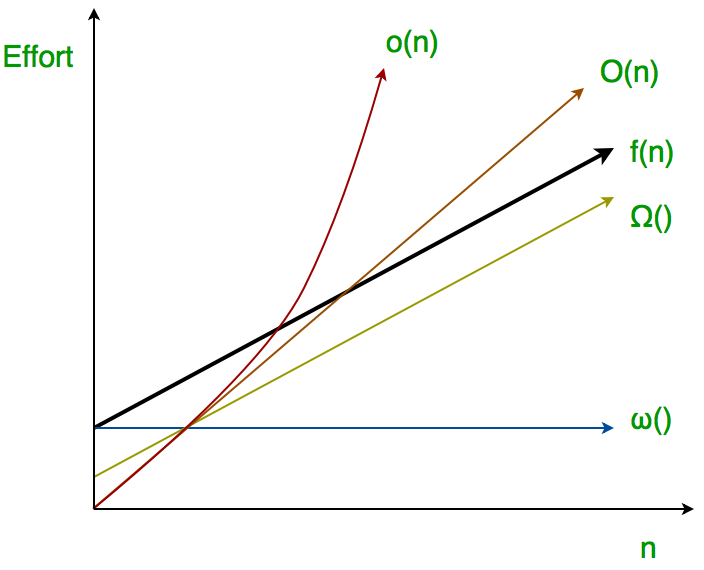GeeksforGeeks App
Open AppBrowser
Continue

# Analysis of algorithms | little o and little omega notations

The main idea of asymptotic analysis is to have a measure of the efficiency of algorithms that don’t depend on machine-specific constants, mainly because this analysis doesn’t require algorithms to be implemented and time taken by programs to be compared. We have already discussed Three main asymptotic notations. The following 2 more asymptotic notations are used to represent the time complexity of algorithms.

Little ο asymptotic notation

Big-Ο is used as a tight upper bound on the growth of an algorithm’s effort (this effort is described by the function f(n)), even though, as written, it can also be a loose upper bound. “Little-ο” (ο()) notation is used to describe an upper bound that cannot be tight.

Definition: Let f(n) and g(n) be functions that map positive integers to positive real numbers. We say that f(n) is ο(g(n)) (or f(n) Ε ο(g(n))) if for any real constant c > 0, there exists an integer constant n0 ≥ 1 such that 0 ≤ f(n) < c*g(n).Thus, little o() means loose upper-bound of f(n). Little o is a rough estimate of the maximum order of growth whereas Big-Ο may be the actual order of growth.
In mathematical relation, f(n) = o(g(n)) means lim  f(n)/g(n) = 0 n→∞

Examples:

Is 7n + 8 ∈ o(n2)?

In order for that to be true, for any c, we have to be able to find an n0 that makes

f(n) < c * g(n) asymptotically true.

lets took some example,

If c = 100,we check the inequality is clearly true. If c = 1/100 , we’ll have to use

a little more imagination, but we’ll be able to find an n0. (Try n0 = 1000.) From

these examples, the conjecture appears to be correct.

then check limits,

lim  f(n)/g(n) = lim  (7n + 8)/(n2) = lim  7/2n = 0 (l’hospital)

n→∞ n→∞ n→∞

hence 7n + 8 ∈ o(n2)

Little ω asymptotic notation

Definition : Let f(n) and g(n) be functions that map positive integers to positive real numbers. We say that f(n) is ω(g(n)) (or f(n) ∈ ω(g(n))) if for any real constant c > 0, there exists an integer constant n0 ≥ 1 such that f(n) > c * g(n) ≥ 0 for every integer n ≥ n0.

f(n) has a higher growth rate than g(n) so main difference between Big Omega (Ω) and little omega (ω) lies in their definitions.In the case of Big Omega f(n)=Ω(g(n)) and the bound is 0<=cg(n)<=f(n), but in case of little omega, it is true for 0<=c*g(n)<f(n).

The relationship between Big Omega (Ω) and Little Omega (ω) is similar to that of Big-Ο and Little o except that now we are looking at the lower bounds. Little Omega (ω) is a rough estimate of the order of the growth whereas Big Omega (Ω) may represent exact order of growth. We use ω notation to denote a lower bound that is not asymptotically tight. And, f(n) ∈ ω(g(n)) if and only if g(n) ∈ ο((f(n)).

In mathematical relation,

if f(n) ∈ ω(g(n)) then,

lim  f(n)/g(n) = ∞

n→∞

Example:

Prove that 4n + 6 ∈ ω(1);

the little omega(ο) running time can be proven by applying limit formula given below.

if lim  f(n)/g(n) = ∞ then functions f(n) is ω(g(n))

n→∞

here,we have functions f(n)=4n+6 and g(n)=1

lim   (4n+6)/(1) = ∞

n→∞

and,also for any c we can get n0 for this inequality 0 <= c*g(n) < f(n), 0 <= c*1 < 4n+6

Hence proved.